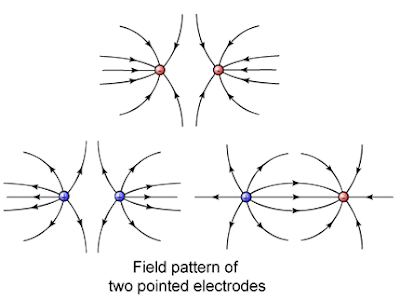## Sunday, 1 April 2012

### Electric Charge and Electric Field

Electric Charge

1.      What is an electric charge? An electric charge is anything that produces an electric field in the space around it. It can be positive or negative.

2.      An electric charge can be: an electron, a proton, a nucleus (an alpha particle i.e. helium nucleus), an ion, a charged molecule or any charged object such as a charged cloud, a plastic comb, an electrode or the charged dome of the Van de Graaff generator:
* Like charges repel
* Unlike charges attract

3.      When there is a flow of electric charge, there is an electric current – this is shown by the “Van de Graaff Generator and Galvanometer” experiment (pg. 43 of F5 textbook).

4.      Electric charge Q is a derived quantity and its unit of measurement coulomb C is a derived unit:

1. The amount of electric charge Q flowing through a point in a circuit is given by the product of current, I, and time of flow of the current, t, across the point, that is:
Q = I x t …………….(Yr 2005 SPM P1 Q42 at pg. 12);
(Yr 2007 SPM P1 Q38 at pg. 100)

Alternatively, Q = ne
(where n = number of electrons or protons; e = charge of an electron or proton)

1. Accordingly (from Q = I x t), electric current is the rate of charge flow of electric charge:
I = Q / t…………….. (Yr 2011 SPM P1 Q35 at pg. 288)

1. Notwithstanding the foregoing, students must know that electric current is a base quantity (i.e. a fundamental physical quantity). And, SI (International System of Units) defined the base unit for electric current ampere, A in terms of its electromagnetic force effect (rather than rate of flow of electric charge), as follows:
One ampere is the constant current which - if maintained in 2 straight parallel conductors of infinite length, of negligible cross-sectional area, and placed 1 metre apart in a vacuum - produces a force of 2 x 10-7 newton per metre of length.”

5.      An electron carries an electric charge e = - 1.6 x 10-19 C. If a steady current of 1 A is flowing for 1 second past a point in a circuit:
1. the electric charge flowing past is Q = I x t = 1 A x 1 s = 1 C. And,
2. therefore, the number of electrons flowing past the point to give 1 C of electric charge = 1 / 1.6(10-19) = 6.25 x 1018 electrons.

Electric Field

1.   What is an electric field? An electric field is the space around a positive or negative electric charge in which any other electric charge would experience an electrical force acting on it.
(Yr 2005 SPM P1 Q45 at pg. 13)

2.      An electric field can be represented by arrowed lines known as the electric field lines or the electric lines of force.

3.      By convention, the direction of electric field lines is from positive to negative – that is, the path that would be taken by a positive test charge if placed in the electric field.

4.      The closer the electric field lines, the stronger the electric force.

5.      An electric field line is a vector quantity because it has magnitude and direction of force.

6.      The pattern of electric field lines depends on the shape and the number of the charged objects in the vicinity (Refer: “Semolina Powder on Olive Oil and Metal Electrodes” experiment for the various patterns – pg.349) (Yr 2008 SPM P1 Q35 at pg. 147)

The Electric Field Pattern:7.      The effect of electric field on a charge can be illustrated by
i.      Polystyrene (Ping-Pong) Ball” Experiment (Click the link to see video showing this) (or, see pg. 350 of Fajar Oxford Reference Book); and,
(Yr 2010 SPM P1 Q33 at pg. 239)
ii.      “Candle Flame” Experiment (pg.351 or pg 46 of F5 textbook).
(Yr 2011 SPM P1 Q37 at pg. 288)
(Yr 2010 SPM P2 CQ12(a) at pg. 264)

Candle Flame in the electric field
• Heat energy from the candle flame produces ionization of air molecules to form positive and negative ions.
• Movement of positive ions which are heavier towards the negative plate causes a bigger spread of the flame.
• Negative electrons which are lighter move towards the positive plate and causing a smaller spread of the flame. (E.H.T. voltage supply means "extra high tension" voltage supply)

-------------------------------------------
Updated by: tutortan1@gmail.com(23/06/16)